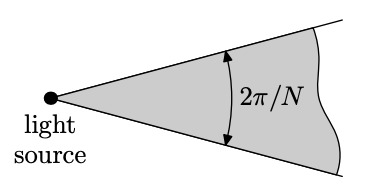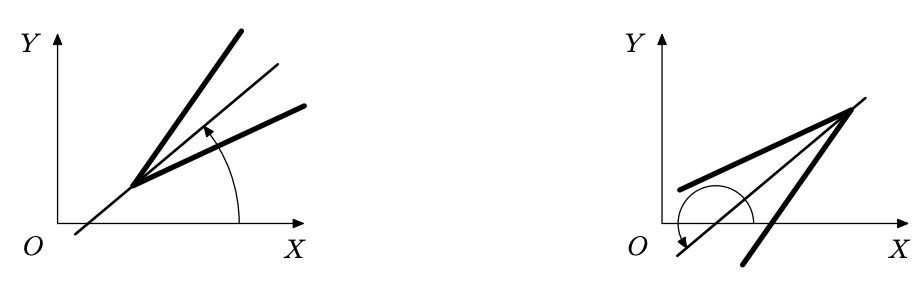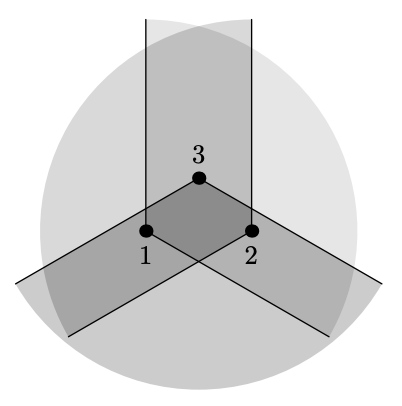시간 제한 메모리 제한 제출 정답 맞은 사람 정답 비율
1 초 128 MB 2 1 1 50.000%

## 문제

You are given $N$ light sources on the plane, each of which illuminates the angle of $2\pi/N$ with the vertex in the source point (including its sides).You must choose the direction of the illuminated angle for each of these sources, so that the whole plane is illuminated. It can be proved that this is always possible.

A light source itself casts no shadow and does not interfere with light beams from the other sources.

## 입력

The first line of the input file contains $N$ --- the number of light sources ($3 \le N \le 30$). Next $N$ lines contain two integer numbers each --- the coordinates of the light sources. All coordinates do not exceed $100$ by their absolute value. No two sources coincide.

## 출력

Print $N$ real numbers --- for each light source specify an angle that the bisector of the illuminated angle makes with $OX$ axis, counterclockwise. Print at least six digits after the decimal point. No angle must exceed $100 \pi$ by its absolute value.## 예제 입력 1

3
0 0
2 0
1 1


## 예제 출력 1

0.52359877559829887
2.61799387799149437
4.71238898038468986


## 힌트